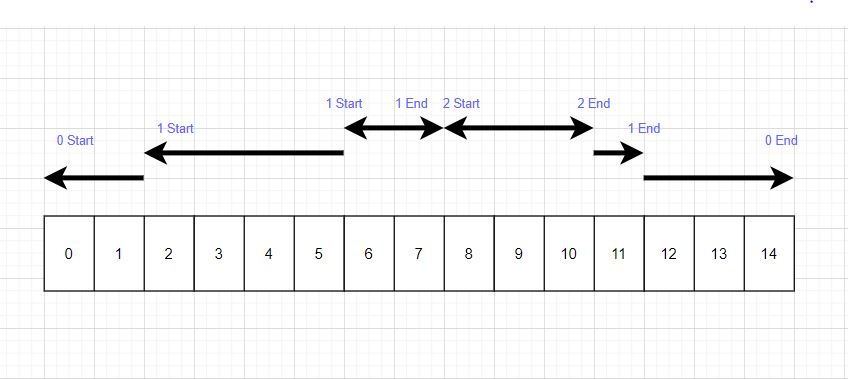0

# Execution Time

Difficulty: MEDIUMContributed By
Mutiur Rehman khan
Avg. time to solve
15 min
Success Rate
85%

Problem Statement

#### You are given a 2D array of integers containing information about ‘L’ logs where ith column represents the ith log message. Each column contains 3 rows to describe the ith log where,

``````1st Row - represents the ID of the function.

2nd Row - represents whether the function has started or ended where 1 denotes the start and -1 denotes the end.

3rd Row - represents the TIMESTAMP of the log.
``````

#### Note:

``````1. The exclusive time of a function is the sum of execution times for all calls of that function.

2. A function can be called multiple times, possibly recursively.

3. No two events will happen at the same time where an event denotes either a start or end of a function call. This basically means no two logs have the same timestamp.

4. Each function has an end log for each start log.
``````

#### For Example:

``````Consider the following input
0 1 1 1 2 2 1 0
1 1 1 -1 1 -1 -1 -1
0 2 5 7 8 10 11 14
````````````Thus, we return [5, 7, 3] as a process with ID 0 has taken 5 units of time and process with ID 1 has taken 7 units of time and process ID 2 has taken 3 units of time. A process’s exclusive time is the sum of exclusive times for all function calls in the program. As process Id 1 has called itself so exclusive time is the sum of exclusive times(5 + 2).
``````
##### Input Format:
``````The first line of input contains a single integer ‘T’, representing the number of test cases or queries to be run.
Then the T test cases follow.

The first line of each test case contains two single space-separated integers ‘N’ and ‘L’, representing the number of unique functions and number of logs respectively.

The second line of each test case contains ‘L’ single space-separated integers representing the function ID for each log.

The third line of each test case contains ‘L’ single space-separated integers(1 or -1), where 1 represent a function that has started execution and -1 represent a function that has ended the execution

The fourth line of each test case contains L single space-separated integers, representing the ‘TIMESTAMP.
``````
##### Output Format:
``````For each test case, print 'N' single space-separated integers, representing the exclusive time of each function in a single line.

The output of each test case will be printed in a separate line.
``````
##### Note:
``````You do not need to print anything, it has already been taken care of. Just implement the given function.
``````
##### Constraints:
``````1 <= T <= 10
1 <= N <= 10 ^ 2
2 <= L <= 5 * (10 ^ 2) and L is even.
0 <= TIMESTAMP <= 10 ^ 9

Time Limit: 1 sec.
``````
##### Sample Input 1:
``````1
2 4
0 1 1 0
1 1 -1 -1
0 2 5 6
``````
##### Sample Output 1:
``````3 4
``````
##### Explanation for Input 1:
``````Function 0 starts at the beginning of timestamp 0, then it executes for 2 units of time and reaches the end of timestamp 1.

Function 1 starts at the beginning of timestamp 2, executes for 4 units of time, and ends at the end of timestamp 5.

Function 0 resumes execution at the beginning of timestamp 6 and executes for 1 unit of time.

So function 0 spends 2 + 1 = 3 units of total time executing, and function 1 spends 4 units of total time executing.
``````
##### Sample Input 2:
``````1
1 6
0 0 0 0 0 0
1 1 -1 1 -1 -1
0 2 5 6 6 7
``````
##### Sample Output 2:
``````8
``````Console